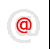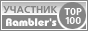Apache-Talk @lexa.ruInet-Admins @info.east.ruSecurity-alerts @yandex-team.runginx-ru @sysoev.ruÈ˚ÈÙÂÈÛ¯Ì··ÚËÈ˜ :: Filmscanners
Filmscanners mailing list archive (filmscanners@halftone.co.uk)

### RE: filmscanners: Magnification of light - AND brief density math lesson...

```Yes, your log numbers make total sense, as usual. :-)

But, as you imply, the theoretical numbers don't completely correspond to
"Real World" performance. Other factors literally enter into the picture.
Noise, for example, and the light/dark "star" (halo) aberations for another.

I guess my question is whether these relate to the function of the CCDs or
the mechanical optics of the scanner. Or for that matter, both?

Best regards--LRA

>From: "Austin Franklin" <darkroom@ix.netcom.com>
>To: <filmscanners@halftone.co.uk>
>Subject: RE: filmscanners: Magnification of light - AND brief density math
>lesson...
>Date: Fri, 15 Jun 2001 23:03:15 -0400
>
>
> > But yes, as I understand it (scanner-wise), DMax is the maximum amount
>of
> > density a CCD will recognize and record from a piece of film as "Black."
> > DMin is the maximum amount of light (non-density) a CCD will recognize
>as
> > "White."  The Density Range is what lies between.
>
>All correct, but that's not the math involved.  Here's a brief density math
>lesson...
>
>1) Density is relative:
>
>Let's call density values, such as DMax, "D" values.  Density is described
>as a ratio value...ie, relative to something else.
>
>As a note, the "D" values are in Bels, like deci-bels, a decibel is 1/10th
>of a Bel...so a DMax of 3.4 is actually 34dB...  Bels are used to describe
>a
>logarithmic relative power value, and since that is what we are
>measuring...the power of the light, it is fitting.  As far as relating this
>to audio dB, sound is different than light, so it's not quite the same...
>
>2) Relative to what:
>
>Say you assign the brightest measurement you sense a ratio value of 1:1.
>Simply, the brightest spot is equal to how bright it is.  Something that is
>half as bright is a 2:1 ratio, something that is 1/10th as bright is a 10:1
>ratio...
>
>3) Converting D values to actual ratio values:
>
>A D value of 0 is 10 to the 0th power, which is 1, so the ratio value for a
>D value of 0 is 1:1.  A D value of 1 is 10**1 or a ratio value of 10:1, D
>value of 2 is 10**2 or 100:1, D value of 3 is 10**3 or 1000:1.  A D value
>of
>3.4 is 10**3.4 or a ratio value of ~2,512:1...etc.
>
>A DMax of 3.4, with a DMin of 0, means the scanner can detect a range of
>light intensities from 0, to a value that is 1/2,512th as bright...
>
>4) How does this get related to the numbers of bits my scanner is:
>
>The integer ratio values are what your scanner gives you.  14 bits means
>you
>can represent an integer number from 0 to 16,383.  This means if your
>scanner can record integer density ratio values from 1:1 (0) to 16,384:1
>(16,383).  16,384:1 is a D value of 4.2 (log 16,384 = 4.2).  That is why
>scanners that have 14 bit A/Ds claim to have a DMax of 4.2...all that means
>is they can support integer density ratio values of 1:1 to 16384:1, but
>that
>does not mean the scanner CCD and analog circuitry can provide that!
>That's
>another topic of discussion...
>
>
>That's the simple math behind "D" values and "density ratios".  I'll stop
>there and see if that makes sense...
>

_________________________________________________________________Copyright © Lexa Software, 1996-2009.